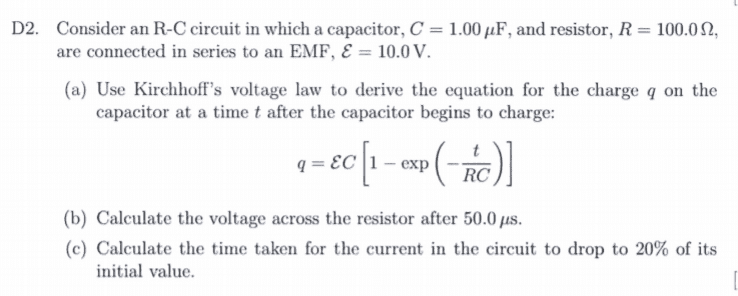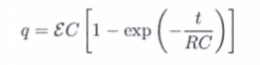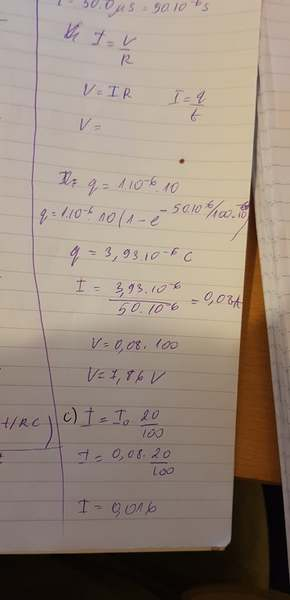# Help with Homework: Part a) Proven, Part b) Checked, Part c?) Stumped

• Jozefina Gramatikova

## Homework Statement## Homework Equations## The Attempt at a SolutionI proved part a)
Can you please check if part b) is correct?
I don't know what to do in part c)

#### Attachments

For part (c), the current is the same everywhere in a series circuit, and can be computed by ## i=\frac{dq}{dt} ## when there is a capacitor in the circuit, where ##q ## is the charge on the capacitor. This can also be used for part b. (Your part (b) is incorrect). Alternatively, you could compute ## V_R=\mathcal{E}-V_C ## where ## V_c=\frac{q}{C} ##.

Last edited:
I get the feeling that you are trying to use some equations without fully understanding them.
1. Why do you think the equation I = q/t is right?
2. I do not see your attempt at part (a). That part is crucial to the understanding of the problem.
3. I do not understand your first value for q, which looks like q = 1 10^(-6) . 10
4. Then there is a second value for q = 3.93 10^(-6) C. Why did you use the same symbol for two different values?
5. The way you got the value for I = 0.08 A is wrong. That equation for I is not valid.